• 图像取反相当于取底片，对于每个像素点的RGB来讲，就是: R′=255−RR'=255-RG′=255−GG'=255-GB′=255−BB'=255-B用R’，G’，B’来替换R，G，B。直接上图吧，假如原图像是这样的： 那么处理后的图像就是这样的...
图像取反相当于取底片，对于每个像素点的RGB来讲，就是: R′=255−R<!--//--><![CDATA[//><!--
R'=255-R
//--><!]]>G′=255−G<!--//--><![CDATA[//><!--
G'=255-G
//--><!]]>B′=255−B<!--//--><![CDATA[//><!--
B'=255-B
//--><!]]>用R’，G’，B’来替换R，G，B。

直接上图吧，假如原图像是这样的：那么处理后的图像就是这样的：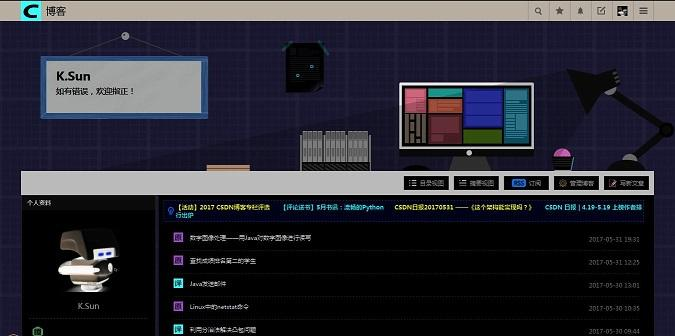代码实现如下：

import java.io.File;
import java.io.IOException;
import java.awt.image.BufferedImage;
import javax.imageio.ImageIO;

public class ImageNegative {
public static void main(String args[]) throws IOException {
BufferedImage image = null;
File f = null;

try {
f = new File("E:\\In.jpg");

int width = image.getWidth();
int height = image.getHeight();

for (int j = 0; j < height; j++) {
for (int i = 0; i < width; i++) {
int p = image.getRGB(i, j);
int a = (p >> 24) & 0xff;
int r = (p >> 16) & 0xff;
int g = (p >> 8) & 0xff;
int b = p & 0xff;

r = 255 - r;
g = 255 - g;
b = 255 - b;

p = (a << 24) | (r << 16) | (g << 8) | b;
image.setRGB(i, j, p);
}
}

f = new File("E:\\Out.jpg");
ImageIO.write(image, "jpg", f);
} catch (IOException e) {
e.printStackTrace();
}
}
}
展开全文java
• 内容有文件（打开、保存、退出）、点处理（彩色转灰阶、图像取反、图像旋转、图像镜像、均衡化、直方图、亮度变化、直方图扩展）、空频域变换（傅里叶变换、傅里叶反变换、离散余弦变换、离散余弦反变换、巴特沃斯低...
• 2、对数、指数变换、取反等各种非线性变换的效果对比，务必有仿真结果及其详细的对比分析 二、1、已知某灰度图像数据如下，请对其进行直方图均衡化 2、已知某灰度图像数据如下，请用Gml和SML两种映射规则对其进行...
实验内容
一、自选一幅背景较复杂、前景目标较多的交通图像，编程实现如下内容，并写出实验报告。
1、分段线性变换各种情况的变换效果对比
2、对数、指数变换、取反等各种非线性变换的效果对比，务必有仿真结果及其详细的对比分析
二、1、已知某灰度图像数据如下，请对其进行直方图均衡化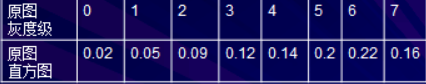2、已知某灰度图像数据如下，请用Gml和SML两种映射规则对其进行直方图规定化，对比变换后的直方图。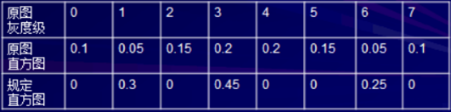1.1线性变换代码：
由于给出的图像是3维RGB彩色图像，故在读取图像后可分为三个方向分别对图像进行操作，即A = I1(:,:,1);B = I1(:,:,2);C = I1(:,:,3);
然后可调用size()获取图像的矩阵像素大小，接着用for循环分别在行和列方向进行遍历操作，分析每一个像素点的大小，将0-255的灰度级分为三个区域，将0-100的灰度级像素点全部变为灰度级为1，将126-255的灰度级像素点全部变为灰度级为254，然后将100-125直接的灰度级像素点变为原像素点的二倍，即将该区域的灰度级进行拓展放大。
clear all
subplot(2,2,1),imshow(I1),title('原图');

A = I1(:,:,1);
subplot(2,2,2),imhist(A),title('原图直方图');
B = I1(:,:,2);
C = I1(:,:,3);
[rows , cols , colors] = size(I1);
for i=1:rows
for j=1:cols
if A(i,j)<=100
A(i,j)=1;
else if A(i,j)>100&A(i,j)<=125
A(i,j)=mod((A(i,j)*2),255);%取余
else
A(i,j)=254;
end
end
end
end
for i=1:rows
for j=1:cols
if B(i,j)<=100
B(i,j)=1;
else if B(i,j)>100&B(i,j)<=125
B(i,j)=mod((B(i,j)*2),255);
else
B(i,j)=254;
end
end
end
end
for i=1:rows
for j=1:cols
if C(i,j)<=100
C(i,j)=1;
else if C(i,j)>100&C(i,j)<=125
C(i,j)=mod((C(i,j)*2),255);
else
C(i,j)=254;
end
end
end
end
D(:,:,1)=A;
D(:,:,2)=B;
D(:,:,3)=C;
subplot(2,2,3),imshow(D),title('分段后的图')
subplot(2,2,4),imhist(A),title('分段直方图')


变换后的图像以及直方图如下图所示：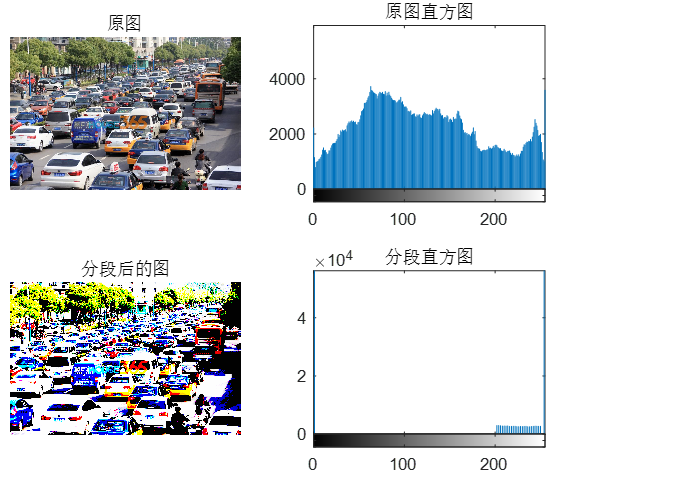由变换后的直方图可以看出，灰度级为1和254的像素点个数非常多，将原图比较均衡的直方图分段线性变换后可得到自己感兴趣灰度级放大后的图像。
1.2指数变换
指数变化与分段线性变换相似，不同点是指数变换是对整体每个像素点的灰度级进行统一的取指数变换，将整体像素点的灰度值进行变换得到新的图。
clear all
I1=im2double(I2);
I1=im2double(I2);
subplot(2,3,1),imshow(I1),title('原图','fontsize',16)
A = I1(:,:,1);
B = I1(:,:,2);
C = I1(:,:,3);
subplot(2,3,4),imhist(A),title('原图直方图','fontsize',16)
[rows , cols , colors] = size(I1);
for i=1:rows
for j=1:cols
A2(i,j)=A(i,j)^2;
B2(i,j)=B(i,j)^2;
C2(i,j)=C(i,j)^2;
A1(i,j)=A(i,j)^4;
B1(i,j)=B(i,j)^4;
C1(i,j)=C(i,j)^4;
end
end
D1(:,:,1)=A1;
D1(:,:,2)=B1;
D1(:,:,3)=C1;
D2(:,:,1)=A2;
D2(:,:,2)=B2;
D2(:,:,3)=C2;
subplot(2,3,2),imshow(D2),title('取以2为底的指数','fontsize',16)
subplot(2,3,5),imhist(A2),title('取对数后的直方图','fontsize',16)
subplot(2,3,3),imshow(D1),title('取4为底的对数','fontsize',16)
subplot(2,3,6),imhist(A1),title('取对数后的直方图','fontsize',16)

观察得到变换后的图像及直方图如图所示：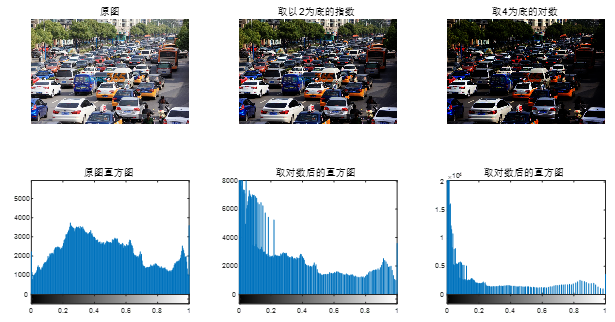由结果可以看出，随着指数的不断增大，图像趋于灰度级0的像素点个数越来越多，图像变得越来越暗。
1.3对数变换：
对数变换与指数变换方法类似，对其整体像素点的灰度值进行取对数变换
clear all
I1=im2double(I2);
subplot(2,3,1),imshow(I1),title('原图','fontsize',16)
A = I1(:,:,1);
B = I1(:,:,2);
C = I1(:,:,3);
subplot(2,3,4),imhist(A),title('原图直方图','fontsize',16)
[rows , cols , colors] = size(I1);
for i=1:rows
for j=1:cols
A1(i,j)=log10(A(i,j)+1);
B1(i,j)=log10(B(i,j)+1);
C1(i,j)=log10(C(i,j)+1);
A2(i,j)=log(A(i,j)+1)/log(5);
B2(i,j)=log(B(i,j)+1)/log(5);
C2(i,j)=log(C(i,j)+1)/log(5);
end
end
D1(:,:,1)=A1;
D1(:,:,2)=B1;
D1(:,:,3)=C1;
D2(:,:,1)=A2;
D2(:,:,2)=B2;
D2(:,:,3)=C2;
subplot(2,3,2),imshow(D2),title('取5为底的对数','fontsize',16)
subplot(2,3,5),imhist(A2),title('取对数后的直方图','fontsize',16)
subplot(2,3,3),imshow(D1),title('取10为底的对数','fontsize',16)
subplot(2,3,6),imhist(A1),title('取对数后的直方图','fontsize',16)

得到变换后的图像及直方图如图所示：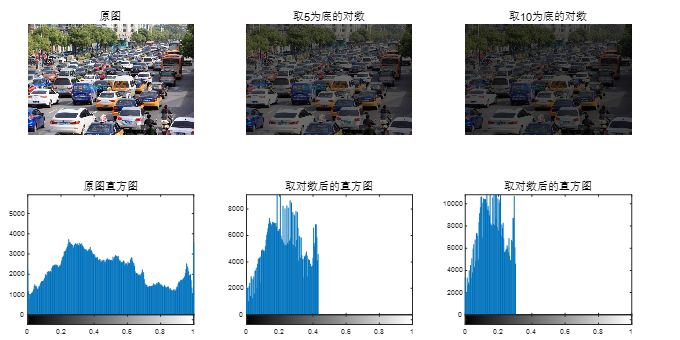观察结果发现图像越来越趋于0，图像变得越来越暗，增强一副图像中较暗部分的细节。
1.4取反变换：
clear all
A = I1(:,:,1);
B = I1(:,:,2);
C = I1(:,:,3);
subplot(2,2,1),imshow(I1),title('原图','fontsize',16)
subplot(2,2,2),imhist(A),title('原图直方图','fontsize',16)
[rows , cols , colors] = size(I1);
for i=1:rows
for j=1:cols
A(i,j)=255-A(i,j);
B(i,j)=255-B(i,j);
C(i,j)=255-C(i,j);
end
end

D(:,:,1)=A;
D(:,:,2)=B;
D(:,:,3)=C;
subplot(2,2,3),imshow(D),title('取反','fontsize',16)
subplot(2,2,4),imhist(A),title('取反直方图','fontsize',16)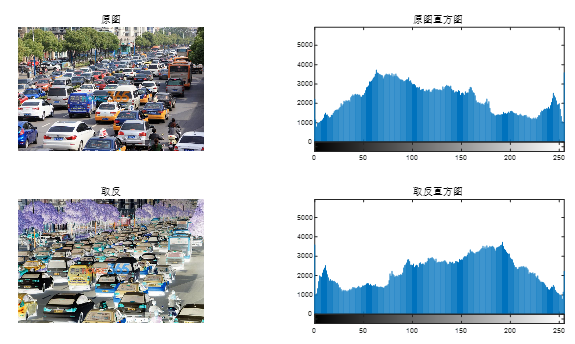其直方图与原图刚好对称。
2.1图像均衡化
先将图像转换为二维的灰度图，再用for循环统计每个灰度级点的个数，并进行归一化处理，求得概率值，然后获得累计概率值，并进行相应的均衡化变化，然后分别将灰度值为0-255直接的每个像素点的灰度值改为均衡化后的灰度值，得到均衡化后的图像。
clear all
I1=rgb2gray(I2);    %将彩色图转化为二维的灰度图
subplot(2,2,1),imshow(I1),title('原图','fontsize',16)
[row,col]=size(I1);   %获取图像的像素点个数
a=zeros(1,256);
a1=zeros(1,256);
for i=0:255
a(i+1)=sum(I1(:)==i)/(row*col);  %统计每个灰度级点的个数，
%并进行归一化处理，求得概率值
a1(i+1)=sum(I1(:)==i);
end
subplot(2,2,2),bar(0:255,a1),title('原图','fontsize',16)
b=zeros(1,256);
for i=1:256
for j=1:i
b(i)=b(i)+a(j);   %获得累积概率值
end
end
c=zeros(1,256);
for i=1:256
c(i)=round(b(i)*255);  %进行相应的变化
end
I3=I1;
for i=0:255
I3(find(I1==i))=c(i+1);  %将灰度值为i的像素点的灰度值改为均
%衡化后的灰度值
end
a2=zeros(1,256);
for i=0:255
a2(i+1)=sum(I3(:)==i);
end
subplot(2,2,3),imshow(I3),title('均衡化后的图','fontsize',16)
subplot(2,2,4),bar(0:255,a2),title('均衡化后直方图','fontsize',16)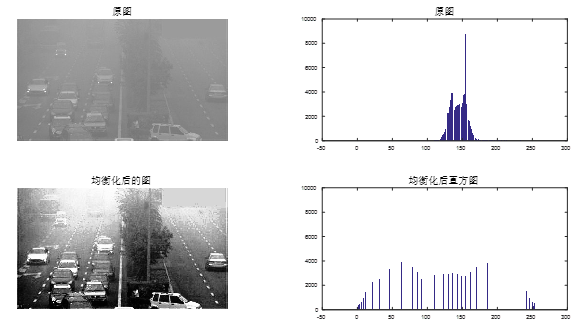由图可以看出，通过图像均衡化处理后，可将原本不清晰的雾天图像变得较为清晰。
图像求均衡化步骤如下：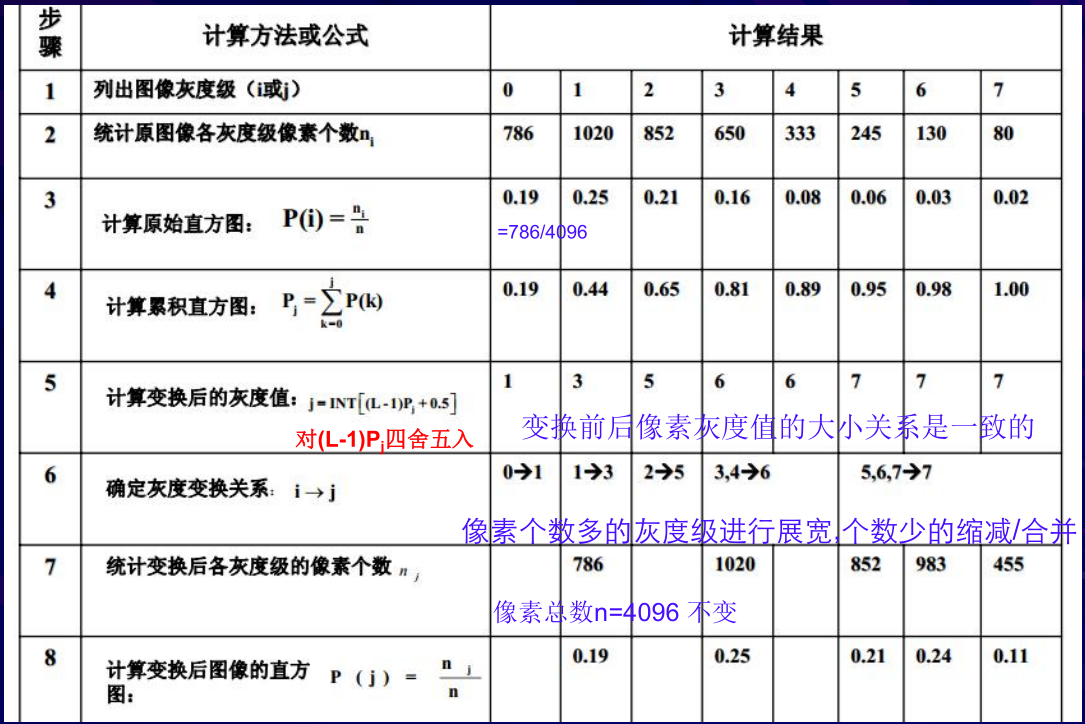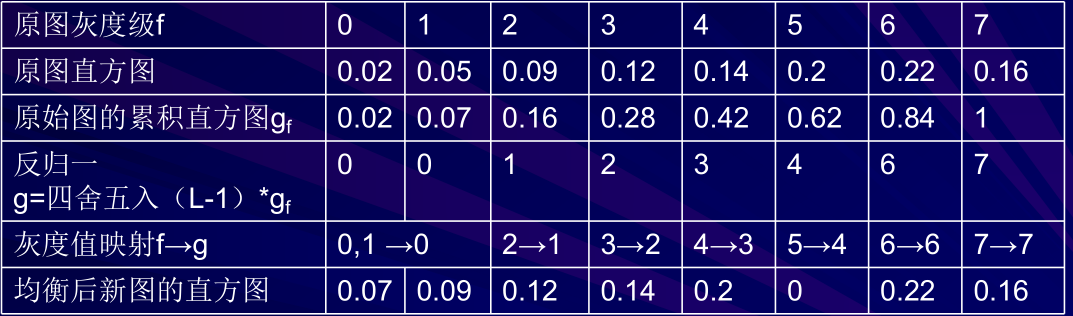2.2直方图规定化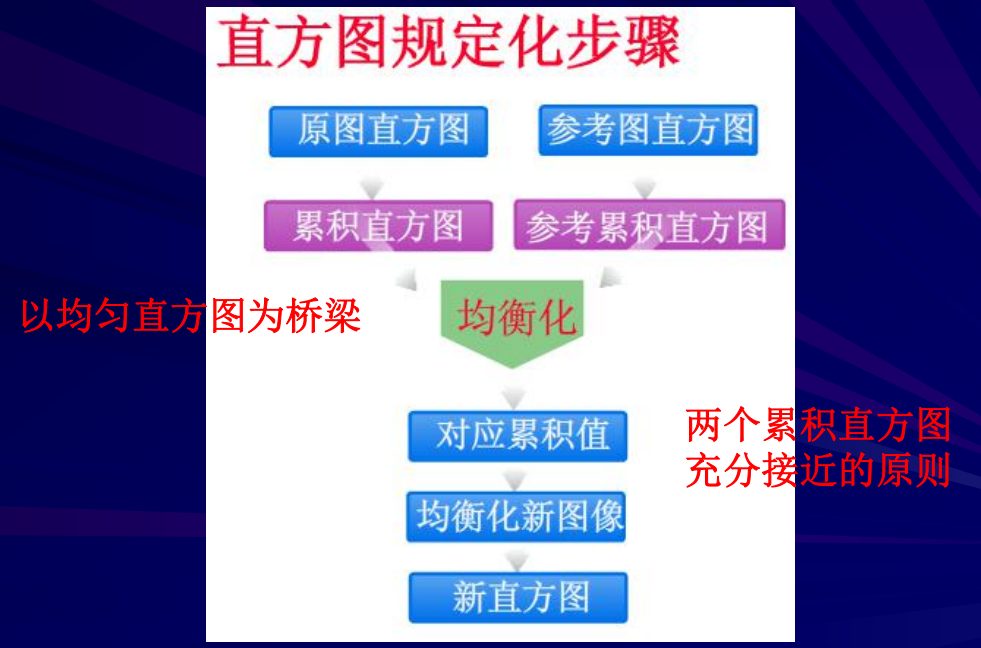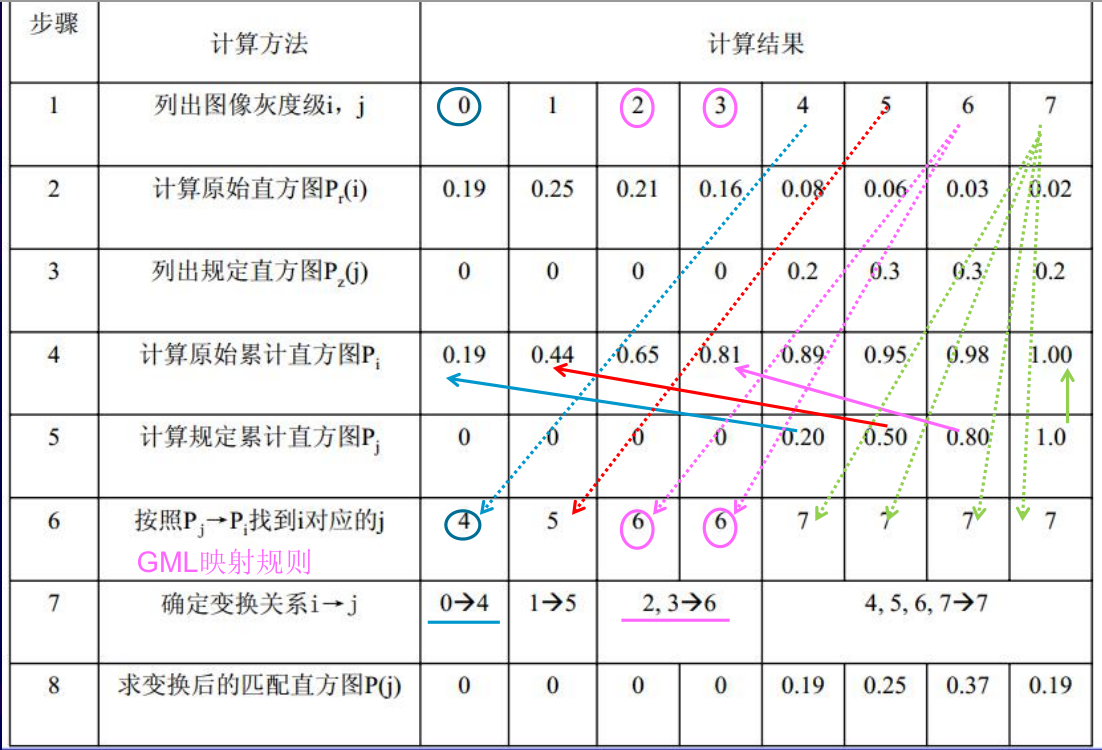以上是GML映射规则，而SML映射与GML恰好相反，SML是从原始图到规定图累积分布概率值的简单的一一对应，满足：累积概率值差 最小的灰度级。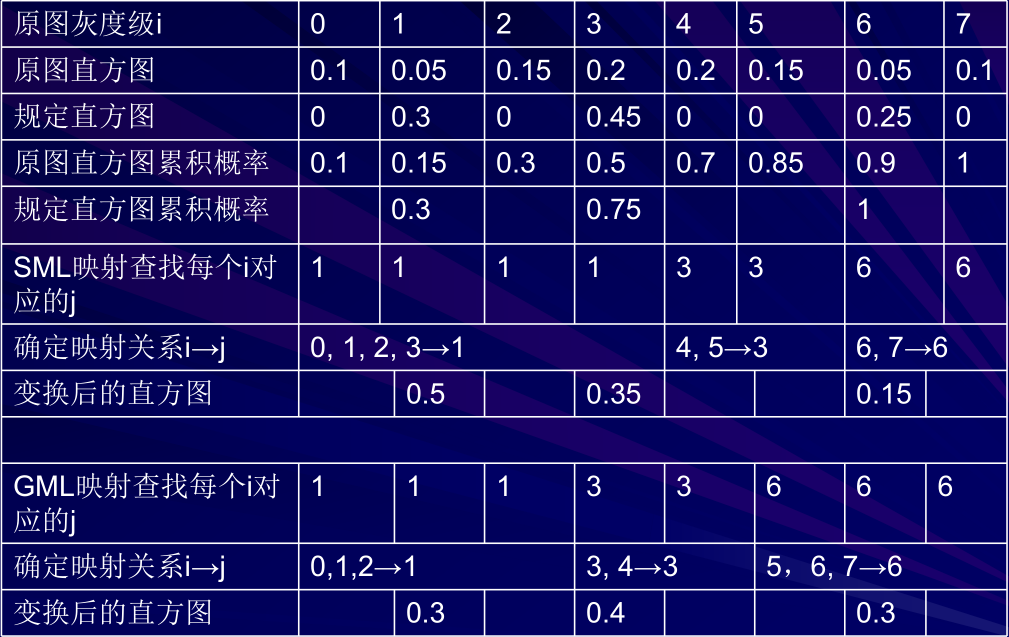展开全文• 包括图像的读入、显示、保存，获取图片大小、通道数等属性，求取图像均值、标准差，遍历图像各个像素，图像取反，图像色彩空间转换，通道分离与合并等 1.基本操作 （1）读入图像 # 默认的读入格式是BGR ...
图像基本操作1.基本操作2.代码示例3.结果展示

包括图像的读入、显示、保存，获取图片大小、通道数等属性，求取图像均值、标准差，遍历图像各个像素，图像取反，图像色彩空间转换，提取某种颜色，通道分离与合并等

1.基本操作

（1）读入图像

# 默认的读入格式是BGR
img1 = cv2.imread('E:/PycharmProjects/one.jpg')

（2）显示图像

cv2.imshow(‘winname’, img)
cv2.waitKey(0)
cv2.destroyAllWindows()
cv2.imshow(winname,  mat) 有窗口名和图片两个参数，不要忘记第一个参数
cv2.waitKey(delay: Any = None) 等待键盘输入。参数为延迟时间，单位为ms。参数为0时表示一直等到有键盘任意输入再进行下一操作。

（3）保存图像

cv2.imwrite('E:/PycharmProjects/one.png', img1)
cv2.imwrite(filename, img) 有文件名和图片两个参数，通过设定文件名的后缀可以转换图片格式

（4）获取图片属性

def img_info(img):
print('type:', type(img))
print('img.size:', img.size)
print('img.dtype:', img.dtype)
print('img.shape', img.shape)
img_info(img1)
type(  )：   获取对象类型，图像类型为numpy.ndarray
.shape： h, w, c 高度、宽度、通道数，最常用
.size： 图像像素总数
.dtype： 数据类型，默认为uint8

（5）图像求均值、标准差

mean_img = cv2.mean(img)
m, stddev = cv2.meanStdDev(img)
图像有三个通道时，结果为三个通道分别求均值和标准差

（6）遍历图像各个像素且取反

import cv2
import numpy as np
def reverse_img(img):
h, w, c = img.shape
r_img = np.ones([h, w, c], dtype=np.uint8)#要注意dtype为np.uint8，否则图像无法正常显示
print(r_img.dtype)
print(r_img)
print(r_img.shape)
for row in range(h):
for col in range(w):
for ch in range(c):
r_img[row, col, ch] = 255-img[row, col, ch]
print(r_img.dtype)
show('reverse_img', r_img)

reverse_img(img1)

（7）图像取反

ot_img1 = cv2.bitwise_not(img1)

（8）图像色彩空间转换

gray = cv2.cvtColor(img, cv2.COLOR_BGR2GRAY)
hsv = cv2.cvtColor(img, cv2.COLOR_BGR2HSV)
HSV（Hue, Saturation, Value）色彩空间，H（色调），S（饱和度），V（明度）

（9）提取图像中某种颜色

low = np.array([0, 0, 0])
up = np.array([255, 100, 100])
blue_img1 = cv2.inRange(img1, low, up)
cv2.inRange(src: Any, lowerb: Any, upperb: Any, dst: Any = None)
lowerb, upperb： numpy.ndarray类型
HSV基本颜色分量范围：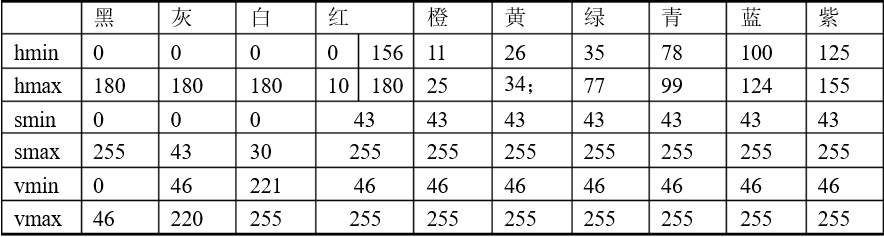（10）通道分离与合并

b, g, r = cv2.split(img)
merge_img = cv2.merge([b, g, r])
2.代码示例
import cv2
import numpy as np

# 1.读入图片和保存图片

# 2.显示图片
def show(name, img):
cv2.imshow(name, img)
cv2.waitKey(0)
show('img1', img1)

# 3.保存图像
cv2.imwrite('E:/PycharmProjects/one.png', img1)

# 4.获取图片属性
def img_info(img):
print('type:', type(img))
print('img.size:', img.size)
print('img.dtype:', img.dtype)
print('img.shape', img.shape)
img_info(img1)

# 5.图像求均值、标准差
def mean_dev(img):
mean_img = cv2.mean(img)
print('mean', mean_img)
m, stddev = cv2.meanStdDev(img)
print('m', m, '\n', 'StdDev', stddev)
mean_dev(img1)

# 6.遍历图像各个像素且取反(有问题)
def reverse_img(img):
h, w, c = img.shape
r_img = np.zeros([h, w, c])
for row in range(h):
for col in range(w):
for ch in range(c):
r_img[row, col, ch] = 255-img[row, col, ch]
show('reverse_img', r_img)

# reverse_img(img1)

# 7.openCV取反函数
def not_img(img):
not_img = cv2.bitwise_not(img)
show('not_img', not_img)
not_img(img1)

# 8.图像色彩空间转换
def color_cvt_img(img):
gray = cv2.cvtColor(img, cv2.COLOR_BGR2GRAY)
hsv = cv2.cvtColor(img, cv2.COLOR_BGR2HSV)
show('gray', gray)
show('hsv', hsv)
color_cvt_img(img1)

# 9.对图片的某种颜色进行提取
def color_inrange(img):
low = np.array([0, 0, 0])
up = np.array([255, 100, 100])
blue_img1 = cv2.inRange(img1, low, up)
show('blue_img1', blue_img1)
color_inrange(img1)

# 10.通道分离、合并
def img_channel(img):
b, g, r = cv2.split(img)
show('blue', b)
print('blue.shape', b.shape)
merge_img = cv2.merge([b, g, r])
show('merge_img', merge_img)
img_channel(img1)
3.结果展示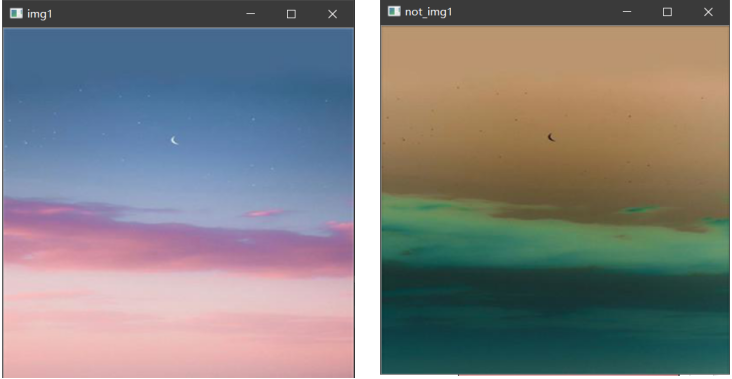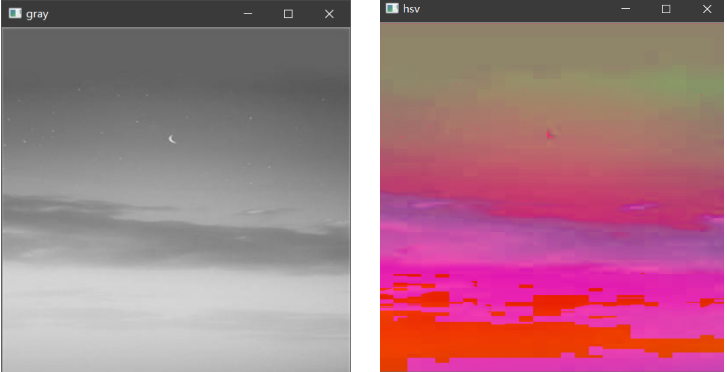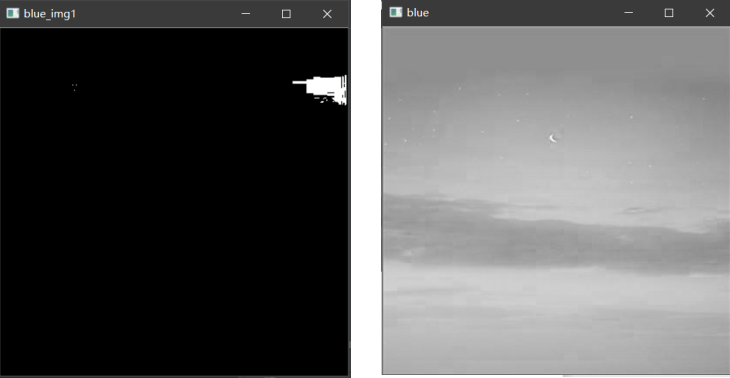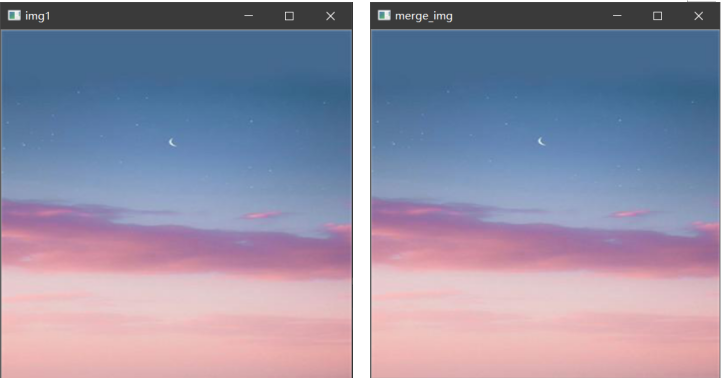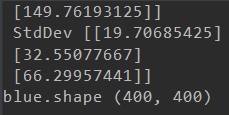展开全文openCV python
• 文章目录一、平台二、实现功能1、打开图片文件2、图像取反3、直方图均衡化4、幂次变换三、遇到问题反思：附代码： 设计一个集成各种图像处理算法的软件（前几次作业已经实现的算法、功能） 一、平台 使用平台：VS...
文章目录一、平台二、实现功能1、打开图片文件2、图像取反3、直方图均衡化4、幂次变换三、遇到问题反思：附代码：
设计一个集成各种图像处理算法的软件（前几次作业已经实现的算法、功能）
一、平台
使用平台：VS2015+MFC
语言：C语言
VS: Microsoft Visual Studio（简称VS）是美国微软公司的开发工具包系列产品。VS是一个基本完整的开发工具集，它包括了整个软件生命周期中所需要的大部分工具，如UML工具、代码管控工具、集成开发环境(IDE)等等。所写的目标代码适用于微软支持的所有平台，包括Microsoft
Windows、Windows Mobile、Windows
CE、.NET Framework、.NET Compact
Framework和Microsoft Silverlight 及Windows Phone。
MFC: 微软基础类库（英语：Microsoft Foundation Classes，简称MFC）是微软公司提供的一个类库（class libraries），以C++类的形式封装了Windows API，并且包含一个应用程序框架，以减少应用程序开发人员的工作量。其中包含大量Windows句柄封装类和很多Windows的内建控件和组件的封装类。
界面如下：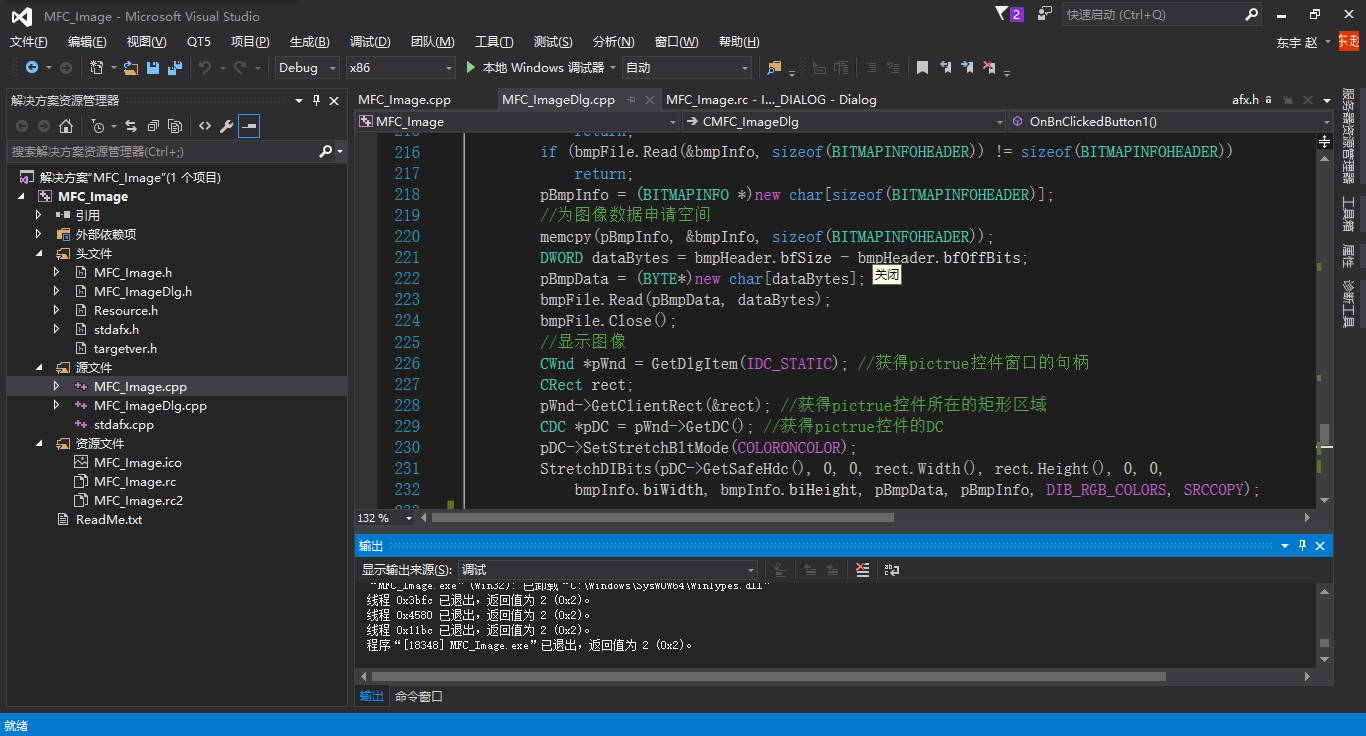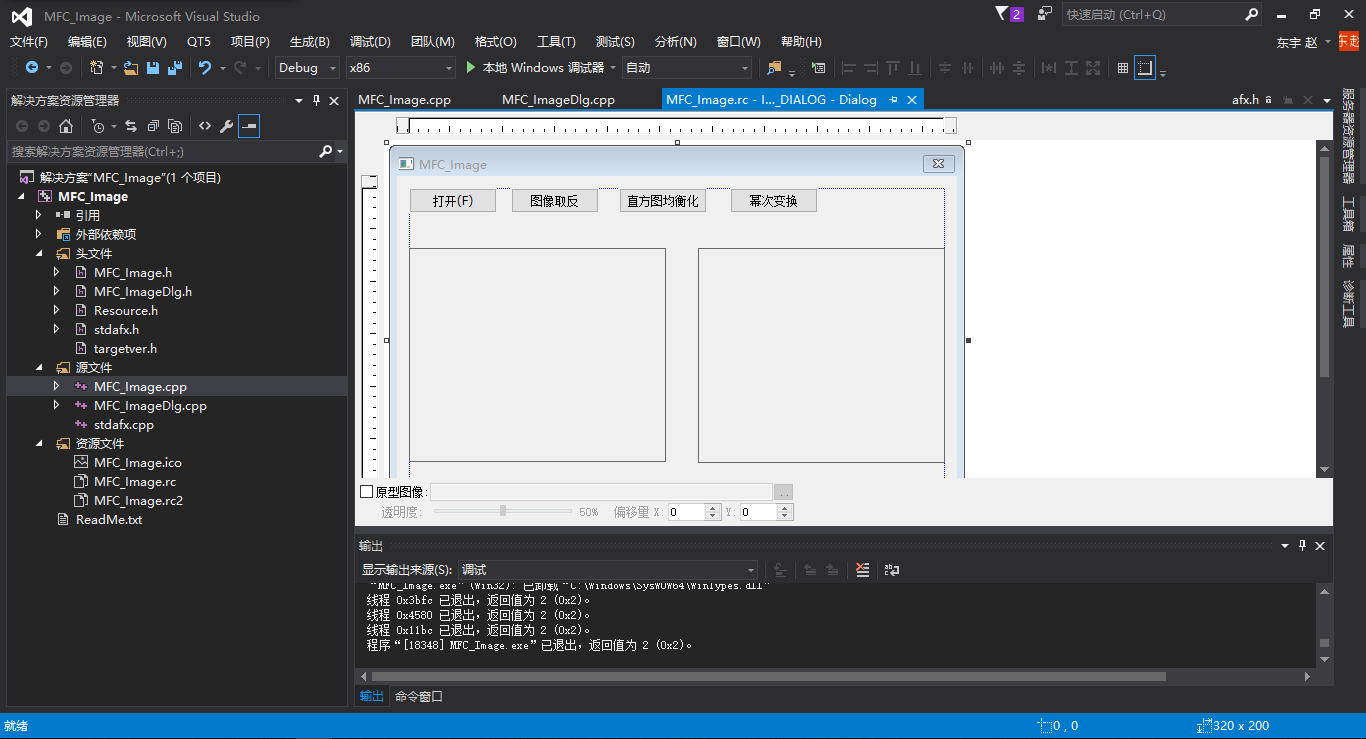二、实现功能
1、打开图片文件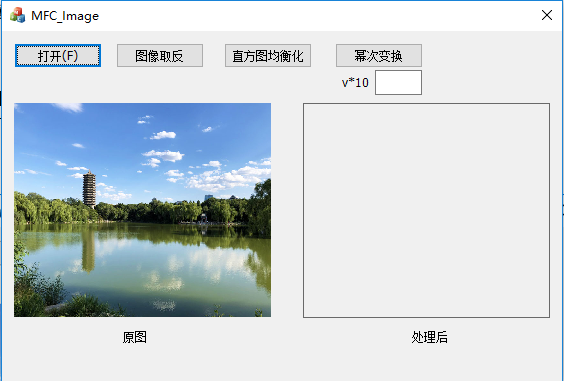点击“打开”按钮，即可选择图片文件进行打开并在左侧窗口显示出来。
目前只支持.bmp格式的图片。
2、图像取反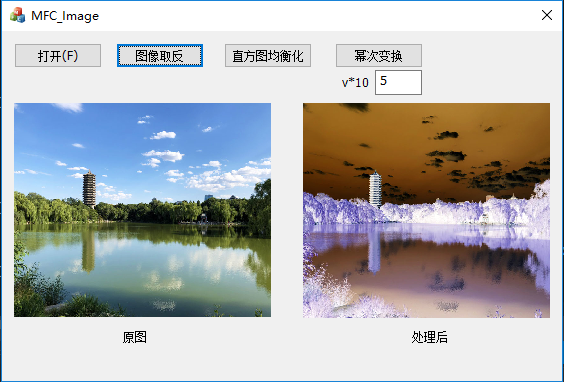点击“图像取反”按钮，可对原图进行取反操作。
3、直方图均衡化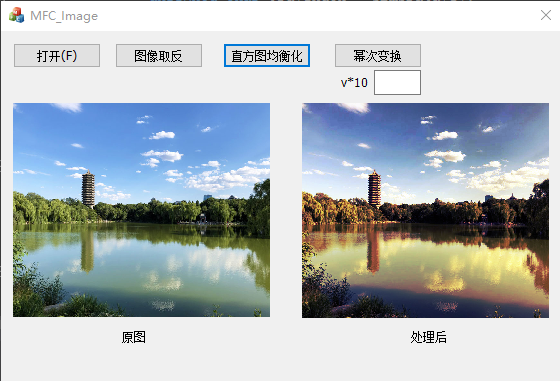（注：这一步的操作是有问题的，彩色直方图均衡化不应该对RGB三通道分别操作，关于这一问题以后会说到，这里就不多说了）
4、幂次变换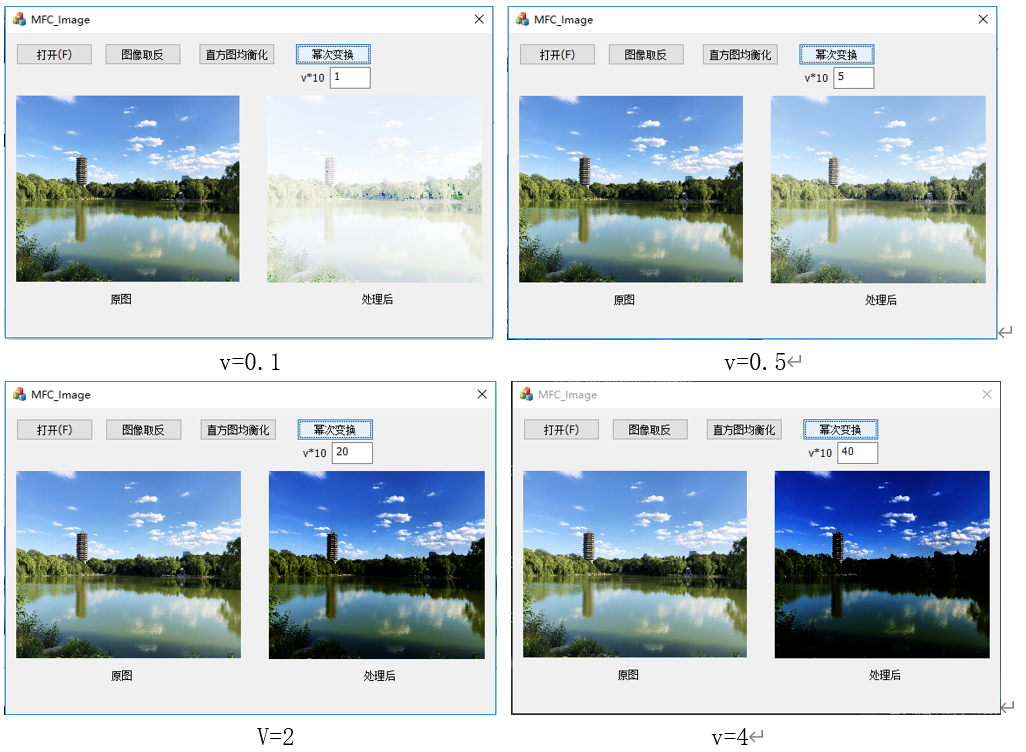输入v*10的值，点击幂次变换按钮实现幂次变换。
三、遇到问题MFC读取的是bmp格式文件，最开始调试时想看一下读取的数据对不对，就把数据输出看了一下，结果如上，左是原图，右是输出的图。可以看到不仅左右上下翻转了，图像颜色也变了。
关于翻转，是因为bmp格式读取图像顺序的原因，这个老师讲过。
关于颜色也变化就于点好奇，，，上网查了一下，原来bmp格式不是RGB格式而是BGR这个顺序。
不过还挺好看的:)
反思：
这周的作业，前段时间一直在纠结用MFC还是QT,最后先学会了MFC便用了MFC。写的代码架构不太好，有点乱。
附代码：
代码有点长，还是之后有时间上传到github吧，到时候会在这里更新地址


展开全文• 处理图像时，会经常对像素进行操作，实时性要求较高的场所往往会使用并行处理，好在（C/C++ API）支持多种并行方式：mpi，openmp，intel ipp 等，今天记录一种利用 openmp简单的并行处理图像方法：灰度图像取反。...
• 二值的形态学图像处理 首先先看两种不同的数学集合操作 取反操作 Ac={x∣x∉A}A^c = \lbrace x | x\notin A\rbraceAc={x∣x∈/​A} 位移操作 Ax={y∣y=a+x,a∈A} A_x = \lbrace y|y=a+x,a\in A \rbrace Ax​=...
• 方法一：分析：这张图片中眼睛非常大，可以先把图片转换成取反的二值图像，先水平投影找到眼睛所在位置的上下坐标，截取出水平长条，再找到长条中所有不为零的像素点的坐标，既得眼睛最左端和最右端的点的坐标。...
• 其基本原理为：(集合X为原二值化图像的像素集合，对X取反求得~X(非X， Y表示), 选择的结构元为s1, 对结构元s1取反的结构元为s2) 首先对用s1对X进行腐蚀得到A1,， 用s2对Y(即~X)进行腐蚀得到A2。最终结果C = A1 & A2...
• 从前面的文字中就可以看出，对数字图像处理都是在像素级上操作的，准确地讲是操作像素点的RGB值，在图像取反和灰度图像转换两篇中已经涉及到了对RGB操作的相关代码，相信大家已经也看到了，就是这一段：for (int j...java
• 基于形态学算法，膨胀后与上取反的原图 算法实现步骤： 1.首先找出所有孔洞的位置，只需知道洞中的一个点的坐标即可，下面直接以改点代替该洞 2.新建一张全零图，用0表示背景，1表示前景，大小与原图相同 （1）取出...
• 除:一幅图像取反和另一幅图像相乘 ????逻辑运算 非 与 或 异或 代数运算——加法 ???? 加法运算的定义 C(x,y) = A(x,y) + B(x,y) ???? 主要应用举例 去除叠加性噪声 生成图像叠加效果 ????去除叠加...
• 在计算机图形学与图像处理中，数字图像的灰度是进行图像识别与处理的基础。我们往往需要先读取数字图像的灰度，然后对其进行分析与处理，如生成灰度直方图、灰度修正、提取图像特征、图像锐化等。本文介绍在Visual J...
• OpenCV图像处理入门学习教程系列，上一篇第一篇：OpenCV2 + 3的安装教程与VS2013的开发环境配置 + JPEG压缩源码分析与取反运算修改...在数字图像处理中，二值图像占有非常重要的地位，首先，图像的二值化有利于图像...OpenCV 二值化
• 【Android】图像像素点理解前言图像处理 -ARGB初始原图AlphaR值测试G值测试B值测试非R值测试非G值测试非B值测试高级图片处理取反Demo 前言 我们知道图像是有像素点组成，那么什么是像素点呢？ 查看一张图片的属性...android
• 110 理解一元运算符：取反运算符和补码运算符 111 理解一元运算符：加法和减法运算符 112 理解算术运算符：倍数运算符(*、/和％) 113 理解算术运算符：加运算符(+和-) 114 理解关系运算符：等于运算符(==和!=) 115 ...
• 110 理解一元运算符：取反运算符和补码运算符 111 理解一元运算符：加法和减法运算符 112 理解算术运算符：倍数运算符(*、/和％) 113 理解算术运算符：加运算符(+和-) 114 理解关系运算符：等于运算符(==和!=) 115 ...